# 有限域和离散对数问题(ECC椭圆曲线算法2)

## 有限域和离散对数问题

The field of integers modulo p

（上面两个例子和原文中不一样，个人觉得原文中举例不恰当。

(
21 mod 4 = 2
2
2 mod 4 = 0
2*3 mod 4 = 2

)

x/y代表什么？数论中，x/y表示 x* y^-1，即 x 乘上 y的逆元。所以除法运算分为两步：第一步求逆元，第二步计算乘法。

def extended_euclidean_algorithm(a, b):
"""
Returns a three-tuple (gcd, x, y) such that
a * x + b * y == gcd, where gcd is the greatest
common divisor of a and b.

This function implements the extended Euclidean
algorithm and runs in O(log b) in the worst case.
"""
s, old_s = 0, 1
t, old_t = 1, 0
r, old_r = b, a

while r != 0:
quotient = old_r // r
old_r, r = r, old_r - quotient * r
old_s, s = s, old_s - quotient * s
old_t, t = t, old_t - quotient * t

return old_r, old_s, old_t

def inverse_of(n, p):
"""
Returns the multiplicative inverse of
n modulo p.

This function returns an integer m such that
(n * m) % p == 1.
"""
gcd, x, y = extended_euclidean_algorithm(n, p)
assert (n * x + p * y) % p == gcd

if gcd != 1:
# Either n is 0, or p is not a prime number.
raise ValueError(
'{} has no multiplicative inverse '
'modulo {}'.format(n, p))
else:
return x % p



Fp上的椭圆曲线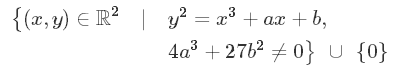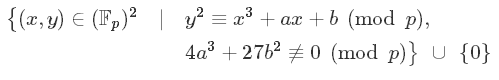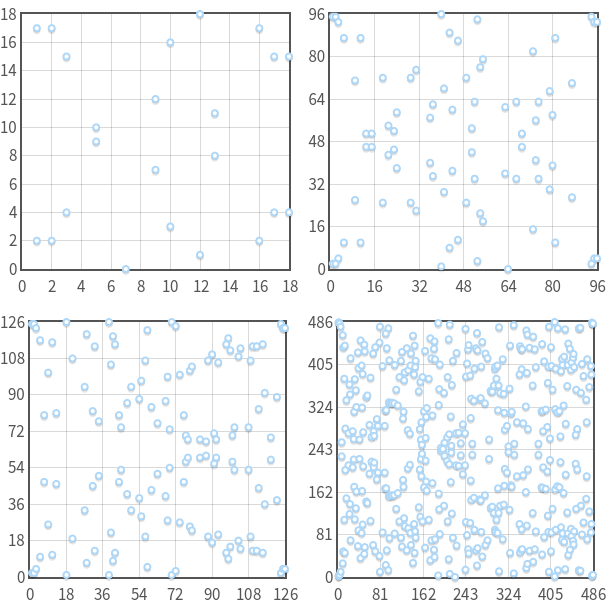Fp上，如果(x, y)满足方程(ax + by+ c ) mod p = 0，则这些(x, y)是共线的.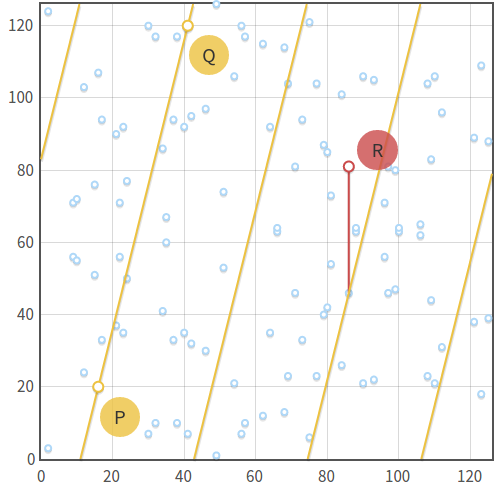(1):Q + 0 = 0 + Q = Q
(2):Q及其逆元-Q，实数域上他两关于X轴对称，但是在Fp上，-Q = (Xq, -Yq mod p)
(3):p + (-P) = 0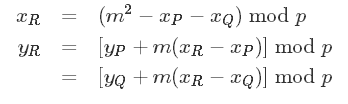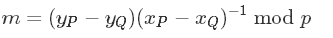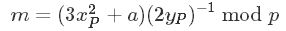https://en.wikipedia.org/wiki/Schoof%27s_algorithm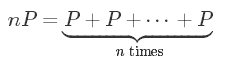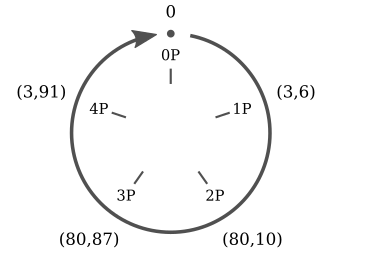nP的取值只有5个不一样的点(0, P, 2P, 3P, 4P)，然后他们周期循环。明显的，椭圆曲线上的数乘和模运算类似：

0P = 0
1P = (3,6)
2P = (80, 10)
3P = (80, 87)
4P = (3, 91)
5P = 0
6P = (3, 6)
7P = (80, 10)
8P = (80, 87)
9P = (3, 91)

5kP = 0
(5k + 1)P = P
(5k + 2)P = 2P
(5k + 3)P = 3P
(5k + 4)P = 4P

kP = (K mod 5) P

1：首先，我们已经定义了阶就是群中点的个数。在子群中也是这样的，但是我们可以换一种表达方式：子群的阶是最小能够使得nP=0的n。就像上文中给出的例子，n是5。
2：子群的阶和群的阶是有关系的。拉格朗日定理说明了，子群的阶是群的阶的因子。即如果N是群的阶，则其子群的阶n，则n|N。

（1）计算群的阶N
（2）找出所有N的因子
（3）每个N的因子n，然后乘以P
（4）在3中，找出最小的n，使得满足nP = 0。则这个n是子群的阶。

1：计算椭圆曲线的阶N。
2：选择一个数n当成子群的阶。n应该是N的素因数
3：计算h = N/n
4：随机选择一个点P
5：计算G = hP
6：如果G是0，到第4步。否则，我们找到了这个基点。

n必须是素数，若非如此，则nP = 0不一定表示n是P的阶，因为P的阶可能是n的一个因子。

ECC有趣的地方就是，它的离散对数问题，看起来比其他的离散对数问题难多了，这也意味着，在椭圆曲线算法中，我们可以使用更小的值k，来达到其他离散对数难题中同样的安全效果。

09-091968
07-2644612-142665
06-07244
06-02273
07-28263
09-01391
01-222025
12-28